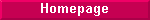﻿ Digital Signal Processing (DSP) - practical introduction for hardware and software engineers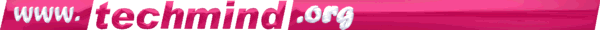by W.A. Steer  PhDDigital Signal Processing (DSP) is a vast and fascinating subject which has exploded in application in recent decades. In its simplest form, high-pass, low-pass, notch, or bandpass filters can be implemented in the digital domain, with far greater precision and stabilty than analog counterparts, and very often at much lower cost. Fourier Transform techniques, impossible in the analogue domain, facilitate highly robust and spectrally-efficient modulation methods used in communications systems such as dial-up modems and digital television and radio broadcasts. State-of-the-art non-linear and data-dependent processing are at the core of all modern audio and video compression systems such as MP3 and MPEG. This page attempts to introduce the key concepts, with practical examples, from an applications, engineering and programming perspective.

Introduction

Digital source data typically consists of a linear-array of 'samples' of some signal collected at uniformly spaced time intervals. Examples include (uncompressed) audio such as .WAV files, and digitised radio-frequency signals. Digital image processing is a whole branch of DSP which deals with two-dimensional (image) datasets, but is not something I intend to dwell on here.

A large part of Digital Signal Processing (DSP) is concerned with frequency-domain processing; this page introduces basic Fourier techniques, concepts of signals, modulation and sidebands, and will demonstrate the methods used for detection and filtering of specific-frequency signals in a dataset (such as DTMF tones in an audio file).

DSP (frequency processing) Basics

Sine waves

Sine-waves are the purest type of waveform, composed of a single frequency. This first section introduces some notation and very basic relationships and identities.

 y(t) = sin(wt)
Maths equation for a basic sine-wave with angular frequency w (angular frequency measured in radians/second).

 y(t) = sin(2πft)
Maths equation for a basic sine-wave with frequency f (frequency measured Hertz, i.e. cycles/second).

 yi = sin(2π·i·f / fs)
DSP equation for discrete sampling-points, i, of a sine-wave with frequency f (in Hertz), in a buffer sampled at a frequency fs samples/second.

Real DSP program-code will usually use the final form of the wave equation in practice.
Beware that for mathematical working, indentities, and proofs, I'll stick with less cumbersome notation!

In physics and electronics we tend to use the phrase 'sine wave' to describe the waveform of a single, pure, frequency without being unduly concerned with its phase. Phase is important in maths and DSP, so we will use 'sin' and 'cos' carefully.

From the standard trigonometric identity:
 sin(A+B) = sinA·cosB + cosA·sinB

we can justify that for a sine wave of arbitrary phase offset, Δ, relative to a reference (such as the start of our buffer):
 sin(φt+Δ) = sin(φt)·cosΔ + cos(φt)·sinΔ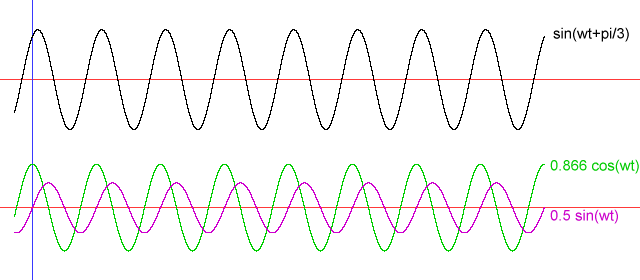Figure 1: Example - sinewave of arbitrary phase analysed as a composite of a sine and cosine 'basis function' waves.

Introducing an arbitrary amplitude, A, for our sinewave, we can generalise this one step further:
 A·sin(φt+Δ) = I·sin(φt) + Q·cos(φt)
where I and Q are the amplitudes of the In-phase (sin) and Quadrature (cos) 'basis function' components respectively.

The phase offset is now implicitly encoded; in this example, Δ = arctan(Q / I)
[In practical programming, avoid using tan and arctan functions as they have a nasty habit of causing overflow or division-by-zero errors!]

The original amplitude is given by:
 A = sqrt(I2+Q2)

Fourier integrals - theory

The basic Fourier integral results tells us that when averaged over an integer number of cycles, the product of two sine (or cosine) terms is ½ if the frequencies are identical, and otherwise zero:

 π { 0 m!=n (1/2π)· ∫ sin mx ·sin nx  dx  = { ½ m=n!=0 -π { 0 m=n=0

 π { 0 m!=n (1/2π)· ∫ cos mx ·cos nx  dx  = { ½ m=n!=0 -π { 1 m=n=0

where m and n are integer.

Also note that the integral product of mixed sin and cos terms always equals zero.
 π (1/2π)· ∫ sin mx ·cos nx  dx  = 0 -π

As we shall see later, these results are the key to being able to detect the presence (amplitude and phase) of single-frequency components in arbitrary digitised signals.

Going one step further, Fourier showed that any arbitrary periodic function f(x) (periodic in {-π,π}) can be analysed as a series of coefficients of sine and cosine harmonic terms:

 f(x) = ½·a0  + a1·cos x + b1·sin x  + a2·cos 2x + b2·sin 2x + a3·cos 3x + b3·sin 3x + ...

 π an = (1/π)· ∫ f(x) ·cos nx  dx -π

 π bn = (1/π)· ∫ f(x) ·sin nx  dx -π

These are absolutely fundamental results for any kind of Digital Signal Processing (DSP) involving detection or analysis of periodic frequencies in data.

Note: a harmonic frequency component in f(x) which has a phase shift such that it is offset from both the sine and cosine analysis functions will contribute to a mixture of both the corresponding an and bn coefficients.
The amplitude of any present component, regardless of phase, can always be calculated as sqrt(an2 + bn2) .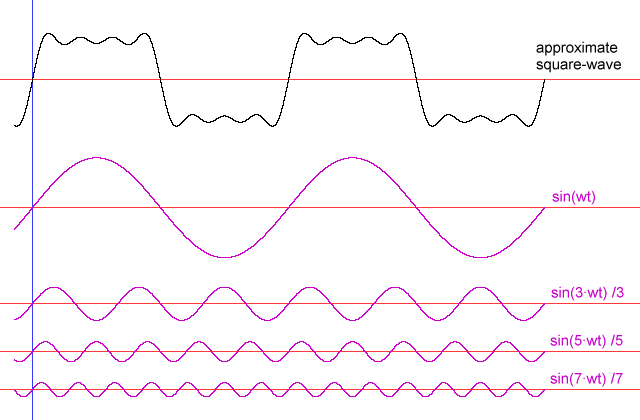Figure 2: Example - square-wave synthesised using Fourier cosine coefficients an = 0, and sine coefficients bn = { (1/n), n odd; 0, n even }

Note: You will often see Fourier integrals and related theory written using e notation, where:

 eiφ = cos φ + i·sin φ

This notation is very compact, and enables both the cosine and sine terms to be expressed in a single equation, but can take some getting used to. In many programming languages you'll need to revert to explicit sin() and cos() for the coding anyway. For that reason, this is the last of e on this page!

Fourier integrals - in practice

If we want to test for the presence of a signal with known frequency, φ, in some data (e.g. a snippet of an audio recording) we need to multiply that data, sample by sample, separately with both cos φt and sin φt . The signal we're looking for has the general equation { A·sin(φt+Δ) } where A is the amplitude, and Δ is the phase offset relative to the start of the buffer. Standard trigonometric identities allow us to describe the result of these multiplications in a more useful form:

 sin(φt) · { A·sin(φt+Δ) } = ½·A·{ cos(Δ) - cos(2φt+Δ) }

 cos(φt) · { A·sin(φt+Δ) } = ½·A·{ sin(Δ) + sin(2φt+Δ) }

and just for completeness...

 sin(φt) · { A·cos(φt+Δ) } = ½·A·{ -sin(Δ) + sin(2φt+Δ) }

 cos(φt) · { A·cos(φt+Δ) } = ½·A·{ cos(Δ) + cos(2φt+Δ) }

When these results are numerically summed over an integer number of cycles, the cos(2φt+Δ) and sin(2φt+Δ) terms average out to zero, leaving:

 ∑ sin(φt) · { A·sin(φt+Δ) } = ½·A· ∑ cos(Δ)   = ½·A·N·cos(Δ)

 ∑ cos(φt) · { A·sin(φt+Δ) } = ½·A· ∑ sin(Δ)   = ½·A·N·sin(Δ)

where N is the number of samples (data-points) summed over.Figure 3: Example - multiplication of example waveform by sin() and cos() basis functions. The steady-state offset is equal to half the magnitude of that component in the original signal, and can be negative.

Windowing and Short-Term Fourier Transforms

Armed the the knowledge above, given some raw digitised (uncompressed) audio data (eg direct from the soundcard, or from a .WAV file), you are almost set to monitor that audio data for DTMF (telephone dialling) tones or specific musical notes. You would however very quickly stumble across a few more issues...
1. Real-world recordings are rarely constant over time; you might have a sequence of telephone-tones, or a piece of music with frequently-changing prominent notes. You will need to analyse a succession of short timeslices of your recording (for audio applications, we typically work with timeslices of a few tens of milliseconds). You need to think about how quickly your detection system will react to changes in the input waveform data
2. The basic Fourier integrals only work properly for periodic signals. Your analysis (summation/integration) interval must be a whole number of cycles of your recorded signal or waveform, as well as a whole number of cycles of your reference (sought) waveform - which in the general practical case will not be so!
3. You need to define somehow how 'precise' your filter/detector algorithm is going to be, what frequency 'tolerance' it should have
We will shortly see that all three problems are interrelated, but let's start by looking at (2) in more detail.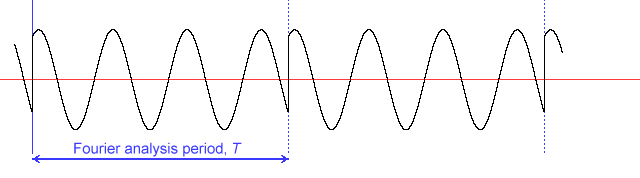Figure 4: A signal frequency which is not periodic in the Fourier analysis period, T, is still 'perceived' by the analysis as cyclical.

A signal frequency which is not periodic in the Fourier analysis period, T, is still 'perceived' by the analysis as cyclical (refer to figure 4). Consequently the analysis typically 'sees' step disjoints in the waveform with a periodicity equal to the analysis period. These disjoints lead to a 'splatter' of rogue harmonics of the analysis period to appear in a full Fourier transform, as well as spurious responses in single-frequency analyses. Further, because we now have an incomplete number of waveform cycles, the average value in the analysis period of this example has become non-zero... we've inadvertently created an apparent DC offset!

A neat solution is to 'window' your source-data. In concept, you gracefully 'fade out' the waveform at each end of your analysis-period. This trick removes the end-effect 'glitches', and introduces a well-behaved pseudo-periodicity.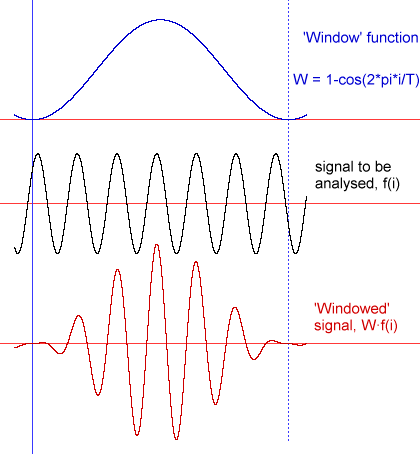Figure 5: 'Windowing' a signal

A commonly-used windowing function is the basic 'raised cosine' window, W=1-cos(2π·i/T) [i is the sample-number, and T is the total number of samples in the window], and is a good starting-point. This window is simple and has the advantage that a time-series of windows can be 50%-overlapped with constant overall amplitude-sensitivity.

You may have observed that a single frequency source signal ( fc ), windowed with the raised cosine function, looks just like an amplitude-modulated (radio) signal, with modulation frequency fm = 1/T samples. Strictly fm = fs· 1/T Hz, where fs is the sampling frequency.

An alternative, and equally valid, intepretation of an AM signal with a fixed modulation frequency, is as a carrier of constant amplitude accompanied by two 'sidebands' with frequencies fc ± fm, each with amplitude equal to one half of the nominal carrier amplitude (if 100% modulation depth to start with).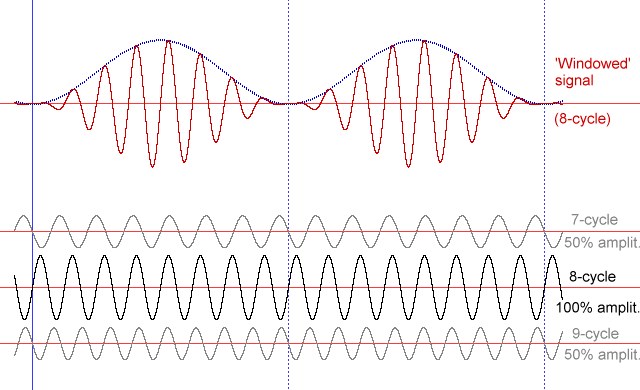Figure 6: Example windowed-signal and its frequency components

Consequently, the raised-cosine-windowed Fourier transform of a sinewave which is periodic in the period of the transform, 'spills' half its amplitude into the two frequency-bins adjacent to that corresponding to the true frequency. This may seem like a bad thing, but the beauty is that the corresponding transform of a sinewave which is not periodic in the transform again only 'spills' relatively locally, rather than contaminating the entire spectrum.

Plenty of alternative window functions are used, with various benefits and drawbacks, and people tend to be very opinionated over which is 'best'. It all depends on the application, but different windows trade-off time- and frequency-resolution, and the exact form of the inevitable 'energy' spillage into adjacent bins (and how much it depends on the distance between the input frequency and the discrete bin-frequencies). Be aware that other windows are available!

A wide window (wide in time, or space) will have a slow response in time, but a sharp (precise) frequency response. A narrow window (narrow in time, or space) will have a faster time response, but a broader frequency response.

Fast Fourier Transform (FFT)

The Fast Fourier Transform is a highly-optimised way of calculating discrete Fourier Transforms for datasets having a length of 2n samples. The workings are well beyond the scope of this page; you simply find an FFT routine from a book (such as Numerical Recipes) and use it! If you require a full transform (as opposed to just extracting one or two specific frequencies) it's almost always worth arranging to work with a 2n sized buffer, eg 256, 512, 1024, 2048, 4096 etc points.

Examples

to be continued (and illustrated)...

References

Most of the trigonometric identities used in the derivations on this page can be found in any (British) 'A'-level maths textbook, such as those by Bostock and Chandler.
You may also find the identities (but with rather less explanation) at www.sosmath.com/

Related pages on this site (www.techmind.org)